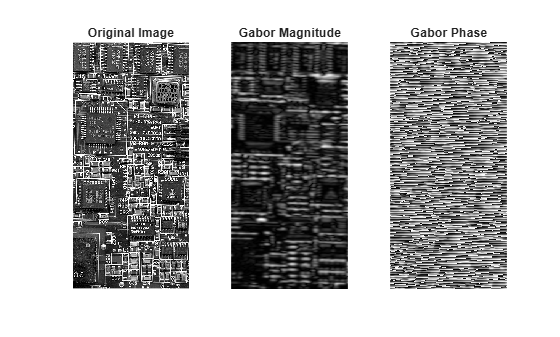# imgaborfilt

Apply Gabor filter or filter bank to 2-D image

## Syntax

``````[mag,phase] = imgaborfilt(A,wavelength,orientation)``````
``[mag,phase] = imgaborfilt(A,wavelength,orientation,Name,Value)``
``````[mag,phase] = imgaborfilt(A,gaborbank)``````

## Description

example

``````[mag,phase] = imgaborfilt(A,wavelength,orientation)``` computes the magnitude and phase response of a Gabor filter for the input grayscale image `A`. `wavelength` describes the wavelength in pixels/cycle of the sinusoidal carrier. `orientation` is the orientation of the filter in degrees.```
````[mag,phase] = imgaborfilt(A,wavelength,orientation,Name,Value)` applies a single Gabor filter using name-value arguments to control various aspects of filtering.```

example

``````[mag,phase] = imgaborfilt(A,gaborbank)``` applies the Gabor filter bank, `gaborbank`, to the input image `A`.```

## Examples

collapse all

Read an image into the workspace and convert the image to grayscale.

```I = imread('board.tif'); I = im2gray(I);```

Apply a Gabor filter to the image.

```wavelength = 4; orientation = 90; [mag,phase] = imgaborfilt(I,wavelength,orientation);```

Display the original image with plots of the magnitude and phase response calculated by the Gabor filter.

```tiledlayout(1,3) nexttile imshow(I) title('Original Image') nexttile imshow(mag,[]) title('Gabor Magnitude') nexttile imshow(phase,[]) title('Gabor Phase')````I = imread('cameraman.tif');`

Create array of Gabor filters, called a filter bank. This filter bank contains two orientations and two wavelengths.

`gaborArray = gabor([4 8],[0 90]);`

Apply filters to input image.

`gaborMag = imgaborfilt(I,gaborArray);`

Display results. The figure shows the magnitude response for each filter.

```figure subplot(2,2,1); for p = 1:4 subplot(2,2,p) imshow(gaborMag(:,:,p),[]); theta = gaborArray(p).Orientation; lambda = gaborArray(p).Wavelength; title(sprintf('Orientation=%d, Wavelength=%d',theta,lambda)); end```## Input Arguments

collapse all

2-D grayscale image, specified as a numeric matrix.

Wavelength of the sinusoidal carrier, specified as a number greater than or equal to 2, in pixels/cycle. Typical values of `wavelength` range from 2 up to the hypotenuse length of the input image .

Orientation of the filter in degrees, specified as a numeric scalar in the range [0, 360]. The orientation is defined as the normal direction to the sinusoidal plane wave.

Gabor filter bank, specified as a `gabor` object or an array of `gabor` objects.

### Name-Value Arguments

Specify optional pairs of arguments as `Name1=Value1,...,NameN=ValueN`, where `Name` is the argument name and `Value` is the corresponding value. Name-value arguments must appear after other arguments, but the order of the pairs does not matter.

Before R2021a, use commas to separate each name and value, and enclose `Name` in quotes.

Example: `'SpatialFrequencyBandwidth',2` specifies a spatial frequency bandwidth of two octaves

Spatial-frequency bandwidth, specified as a numeric scalar in units of octaves. The spatial-frequency bandwidth determines the cutoff of the filter response as frequency content in the input image varies from the preferred frequency, 1/lambda. Typical values for spatial-frequency bandwidth are in the range [0.5, 2.5].

Ratio of the semimajor and semiminor axes of Gaussian envelope (semiminor/semimajor), specified as a positive number. This argument controls the ellipticity of the Gaussian envelope. Typical values for spatial aspect ratio are in the range [0.23, 0.92].

## Output Arguments

collapse all

Magnitude response for the Gabor filter or filter bank, returned as a numeric matrix for a single filter or a numeric array for a filter bank. The p-th plane of `mag` is the magnitude response for the Gabor filter of the same index, `gaborbank`(p).

Data Types: `double`

Phase response for the Gabor filter or filter bank, returned as a numeric matrix for a single filter or a numeric array for a filter bank. The p-th plane of `phase` is the phase response for the Gabor filter of the same index, `gaborbank`(p).

Data Types: `double`

## Tips

• If the image contains `Inf`s or `NaN`s, then the behavior of `imgaborfilt` is undefined because Gabor filtering is performed in the frequency domain.

• For all input data types other than `single`, `imgaborfilt` performs the computation in `double`. Input images of type `single` are filtered in type `single`. Performance optimizations may result from casting the input image to `single` prior to calling `imgaborfilt`.

 Jain, Anil K., and Farshid Farrokhnia. "Unsupervised Texture Segmentation Using Gabor Filters." Pattern Recognition 24, no. 12 (January 1991): 1167–86. https://doi.org/10.1016/0031-3203(91)90143-S.